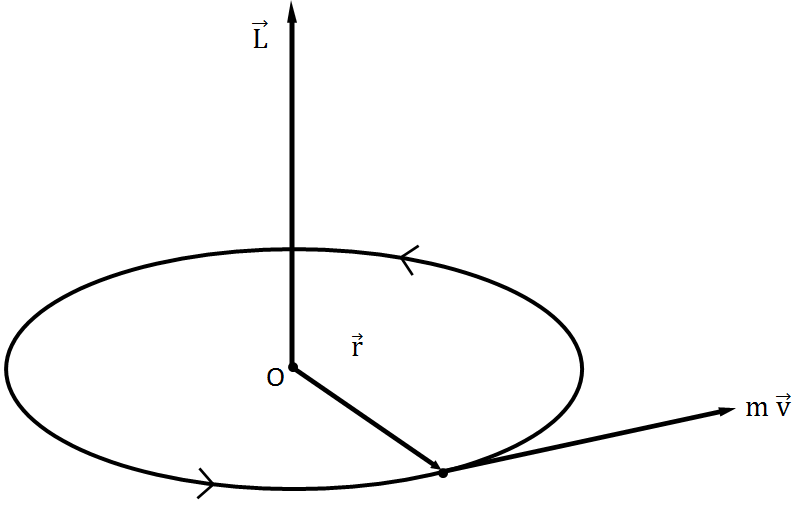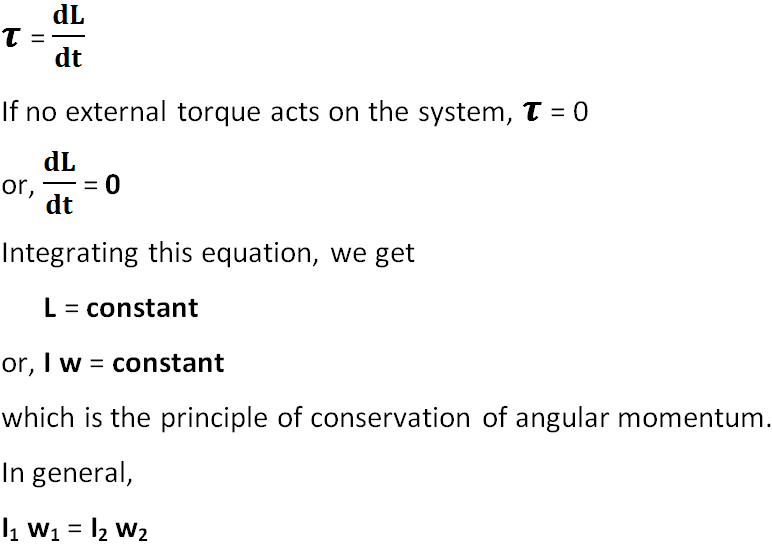# Angular Momentum and Principle of Conservation of Angular Momentum

The moment of linear momentum of an object is called angular momentum. Angular momentum is represented by L.

The angular momentum of an object about an axis of rotation is measured by the product of the linear momentum of the object and the perpendicular distance between the object and the axis of rotation.## Expression for Angular Momentum

Suppose an object of mass m is revolving around a circle of radius r with speed v about an axis passing through center O as shown in the figure.

The linear momentum of the object, p = mv.

The angular momentum of an object, L = linear momentum x perpendicular distance from the axis of rotation.

or, L = p r

Therefore, L = m v r

Since v = w r, where w is the angular velocity of the object, the angular momentum can be written as

L = m (w r) r

or, L = m r2 w

The equations above are the expressions for the angular momentum of the body. It is a vector quantity.

### Angular Momentum Unit and Dimension

In CGS system, the unit of angular momentum is g cm2s-1 and in SI system, its unit is kg m2s-1.

The dimensional formula of angular momentum L is:

L = [M L0 T0] [M0 L T-1] [M0 L T0]

= [M L2 T-1]

## Principle of Conservation of Angular Momentum

Principle of conservation of angular momentum states that,

If no external torque acts on a system, the total angular momentum of the system remains constant.

If I be the moment of inertia of a body about a given axis of rotation and w be its angular velocity, then I w = constant.

This equation represents the mathematical form of principle of conservation of angular momentum.

Proof

We know that the torque acting on a system is equal to the time rate of change of angular momentum of the system about that axis, i.e.Above equation proves that, the angular momentum remains constant if no torque acts on the system.